• 一个例子搞清楚（先验分布/后验分布/似然估计）preface： 无论是《通信原理》还是《概率与统计理论》，或者在现在流行的《模式识别》和《Machine Learning》中总会遇到这么几个概念：先验分布/后验分布/似然估计...
一个例子搞清楚（先验分布/后验分布/似然估计）
preface：

无论是《通信原理》、《信息论》、《信道编码》还是《概率与统计理论》，或者在现在流行的《模式识别》和《Machine Learning》中总会遇到这么几个概念：先验分布/后验分布/似然估计。

如果大家不熟悉这几个词，相信大家熟知贝叶斯公式，该公式涉及到了以上几个概念。但是学完本科课程，也会算题，就是在实际情境中总感觉理不清这几个概念的关系，最近上课老被老师讲的先验、后验搞得晕头转向。因此，如果您和我遇到类似的囧事，这篇文章很适合您。

声明：本文主要内容修改整理于知乎回答1。

本文目标：

一个隔壁小哥的故事故事中的因果和三个概念贝叶斯公式的角色最大似然估计和贝叶斯的关系

隔壁小哥的故事

隔壁小哥要去15公里外的一个公园，他可以选择步行走路，骑自行车或者开辆车，然后通过其中一种方式花了一段时间到达公园。

首先在这个事里边，大家不要关注隔壁小哥去干嘛，也许去送外卖吧：) 。言归正传，这件事中采用哪种交通方式是因，花了多长时间是果。俗话说瓜熟蒂落，皆是因果；因果循环，报应不爽。要理解即将提到的概念，何为因何为果先要搞清楚。

三个概念之后验（知果求因）

隔壁小哥去公园的故事才刚刚开始，假设在这里您已经牢记住这个故事的因和果。故事仍然要接着讲，顺便带出我们的概念。

假设我们已经知道小哥花了1个小时到了公园，那么你猜他是怎么去的（走路or坐车or自行车），事实上我们不能百分百确定他的交通方式，我们正常人的思路是他很大可能是骑车过去的，当然也不排除开车过去却由于堵车严重花了很长时间，当然还有可能他是个赛跑的运动员自己一路飞跑过去的。

假设已经知道小哥花了3个小时才到公园，这个时候我们猜的时候会觉得他很大可能是静静地走路过去的。但是假设已经知道小哥只花了20分钟才到公园，那么正常人会觉得他最大可能是开车奔驰而去。

这种预先已知结果（路上花的时间），然后根据结果估计（猜）原因（交通方式）的概率分布即 后验概率。

例子问题公式化：

P

(

交

通

方

式

∣

花

费

的

时

间

)

P( 交通方式 | 花费的时间 )

修改成一般的公式：

P

(

因

∣

果

)

P( 因 | 果 )

公式正规化：

P

(

θ

∣

x

)

P( \theta | x )

（公式中的 “

∣

|

”读作

g

i

v

e

n

given

，即给定的意思。如

P

(

A

∣

B

)

P(A|B)

即A given B 的概率）

[解释]：看到这里估计大家很奇怪为什么要用

x

x

、

θ

\theta

这样的字母表示，而不是熟悉的

x

x

、

y

y

。这样表示自然是有原因的。在这里大家只需要先暂时记住

θ

\theta

代表因、

x

x

代表果，后面的贝叶斯我们将会具体介绍这些字母的含义。

三个概念之先验概率（由历史求因）

换个情景，我们不再考虑隔壁小哥去公园的结果了。假设隔壁小哥还没去，大早上刚起床，打算吃完早饭再去。

假设我们比较了解小哥的个人习惯，别管怎么了解的：) 。小哥是个健身爱好者就喜欢跑步运动，这个时候我们可以猜测他更可能倾向于走路过去。

当然我的隔壁小哥是个大死肥宅，懒得要命！这个时候我们猜测他更可能倾向于坐车，连骑自行车的可能性都不大。

这个情景中隔壁小哥的交通工具选择与花费时间不再相关。因为我们是在结果发生前就开始猜的，根据历史规律确定原因 （交通方式）的概率分布即 先验概率。

例子问题公式化：

P

(

交

通

方

式

)

P(交通方式)

一般化：

P

(

因

)

P( 因 )

正规化：

P

(

θ

)

P(\theta)

三个概念之似然估计（由因求果）

换个情景，我们先重新考虑隔壁小哥去公园的交通方式。

假设隔壁小哥步行走路去，15公里的路到公园，一般情况下小哥大概要用2个多小时，当然很小的可能性是小哥是飞毛腿，跑步过去用了1个小时左右，极为小的可能是小哥是隐藏的高手，10分钟就轻功跑酷到了。

小哥决定开车，到公园半个小时是非常可能的，非常小的概率是小哥因为途径的路上有车祸堵了3个小时。

这种先定下来原因，根据原因来估计结果的概率分布即 似然估计。根据原因来统计各种可能结果的概率即似然函数。

似然函数问题公式化：

P

(

时

间

∣

交

通

方

式

)

P(时间|交通方式)

一般化：

P

(

果

∣

因

)

P( 果|因 )

正规化：

P

(

x

∣

θ

)

P( x|\theta )

贝叶斯公式

我们熟知的贝叶斯公式是这样的：

P

(

A

∣

B

)

=

P

(

B

∣

A

)

∗

P

(

A

)

P

(

B

)

P( A|B )=\frac{P(B|A) *P(A)}{P(B)}

但在这里我们采用如下形式：

P

(

θ

∣

x

)

=

P

(

x

∣

θ

)

∗

P

(

θ

)

P

(

x

)

P( \theta|x )=\frac{P(x|\theta) *P(\theta)}{P(x)}

后

验

概

率

=

似

然

估

计

∗

先

验

概

率

e

v

i

d

e

n

c

e

后验概率 =\frac{似然估计 *先验概率}{evidence}

[注]：

P

(

x

)

P(x)

即

e

v

i

d

e

n

c

e

evidence

。隔壁小哥去公园很多次，忽略交通方式是什么，只统计每次到达公园的时间

x

x

，于是得到了一组时间的概率分布。这种不考虑原因，只看结果的概率分布即

e

v

i

d

e

n

c

e

evidence

，它也称为样本发生的概率分布的证据。

e

v

i

d

e

n

c

e

evidence

在故事中如下表示：

P

(

时

间

)

P(时间)

或

P

(

果

)

P(果)

知乎回答原文参考 这儿.

深入贝叶斯推断

在这里相信大多数人已经很好地理解了先验概率，后验概率，证据以及和似然估计的概念了。接下来我们将接着讲故事，隔壁小哥到公园以后去做一个游戏，游戏内容如下： 在小哥面前有两个一模一样的宝箱，一号箱子里面有3颗水果糖和1颗巧克力糖；二号箱子里面有2颗水果糖和2颗巧克力糖。 (1) 现在小哥将随机选择一个箱子，从中摸出一颗糖。请问小哥选择一号箱子的概率有多大？ (2) 现在小哥将随机选择一个箱子，从中摸出一颗糖发现是水果糖。请问这颗水果糖来自一号箱子的概率有多大？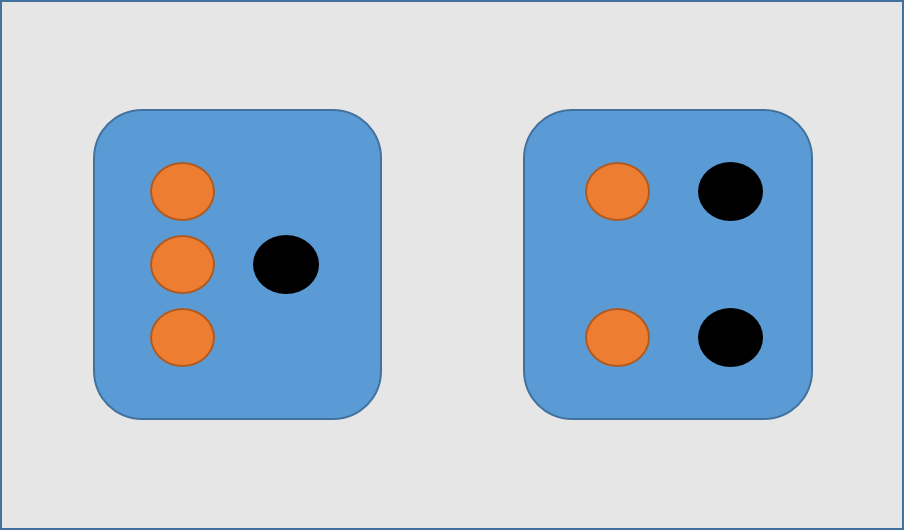暂且不去算这道题，在这个看似无聊的事情中，从哪个箱子去抓是 因；抓到的糖是什么糖为 结果。再去回顾我们之前的贝叶斯公式：

P

(

θ

∣

x

)

=

P

(

x

∣

θ

)

∗

P

(

θ

)

P

(

x

)

P( \theta|x )=\frac{P(x|\theta) *P(\theta)}{P(x)}

[解释]：其中

x

x

是观测得到的结果数据。

P

(

x

)

P(x)

是观测结果数据的概率分布。如下表：

x

x

水果糖巧克力糖

P

(

x

)

P(x)

5/83/8

[解释]：其中

θ

\theta

是决定观测结果数据分布的参数。

P

(

θ

)

P(\theta)

是先验概率，没有观测数据的支持下

θ

\theta

发生的概率。如下表：

θ

\theta

一号箱二号箱

P

(

θ

)

P(\theta)

1/21/2

[解释]：

P

(

θ

∣

x

)

P(\theta|x)

是后验概率，有观测数据的支持下

θ

\theta

发生的概率。在上面的故事中第二问是小哥随机选择一个箱子，从中摸出一颗糖发现是水果糖。这颗水果糖来自一号箱子的概率就是后验概率：

P

(

θ

=

一

号

箱

∣

x

=

水

果

糖

)

P(\theta =一号箱 | x = 水果糖)

[解释]：

P

(

x

∣

θ

)

P(x|\theta)

是似然函数，给定某参数

θ

\theta

时结果数据的概率分布。

其中，

P

(

θ

=

一

号

箱

)

P(\theta =一号箱)

就是先验概率，根据贝叶斯公式，需求证据

P

(

x

=

水

果

糖

)

P(x=水果糖)

和似然函数

P

(

x

=

水

果

糖

∣

θ

=

一

号

箱

)

P( x = 水果糖 | \theta =一号箱)

。

P

(

x

=

水

果

糖

)

=

∑

i

P

(

x

=

水

果

糖

∣

θ

=

i

号

箱

)

P

(

θ

=

i

)

P(x=水果糖) = \sum_{i}P( x = 水果糖 | \theta =i 号箱)P(\theta = i)

我们再考虑上面的计算： (1) 现在小哥将随机选择一个箱子，从中摸出一颗糖。请问小哥选择一号箱子的概率。根据明显的先验知识我们就可以知道

P

(

θ

=

一

号

箱

)

=

1

/

2

P(\theta =一号箱) =1/2

(2) 现在小哥将随机选择一个箱子，从中摸出一颗糖发现是水果糖。请问这颗水果糖来自一号箱子的概率。后验概率为

P

(

θ

=

1

∣

x

=

水

果

)

=

P

(

x

=

水

果

∣

θ

=

1

)

∗

P

(

θ

=

1

)

P

(

x

=

水

果

)

P( \theta =1|x=水果 )=\frac{P(x=水果|\theta =1) *P(\theta =1)}{P(x=水果)}

P

(

θ

=

1

∣

x

=

水

果

)

=

P

(

x

=

水

果

∣

θ

=

1

)

∗

P

(

θ

=

1

)

∑

i

P

(

x

=

水

果

糖

∣

θ

=

i

号

箱

)

P

(

θ

=

i

)

P( \theta =1|x=水果 )=\frac{P(x=水果|\theta =1) *P(\theta =1)}{ \sum_{i}P( x = 水果糖 | \theta =i 号箱)P(\theta = i)}

P

(

θ

=

1

∣

x

=

水

果

)

=

(

3

/

4

)

∗

(

1

/

2

)

(

3

/

4

)

∗

(

1

/

2

)

+

(

2

/

4

)

∗

(

1

/

2

)

P( \theta =1|x=水果 )=\frac{(3/4) *(1/2)}{ (3/4) *(1/2)+(2/4) *(1/2)}

P

(

θ

=

1

∣

x

=

水

果

)

=

3

/

5

P( \theta =1|x=水果 )=3/5

我们为什么要在这里连续计算两道题呢，并不是为了单纯的计算，而是去比较计算结果得到贝叶斯推断的意义。 大家可以看到：没有做实验之前我们推断

P

(

θ

=

一

号

箱

)

=

1

/

2

P(\theta =一号箱) =1/2

这个先验概率；而有了参考结果数据“从中摸出一颗糖发现是水果糖“，我们便可以得到

P

(

θ

=

一

号

箱

∣

x

=

水

果

糖

)

=

3

/

5

P( \theta =一号箱|x=水果糖 )=3/5

这个后验概率。也就是说推断是一号箱的概率，在取出水果糖前和后，【

θ

=

一

号

箱

\theta =一号箱

】事件的可能性得到了增强（

1

/

2

&lt;

3

/

5

1/2 &lt; 3/5

）。

我们可以用小哥在公园的第二个奇遇来解释【贝叶斯估计】的意义： 小哥在公园里玩飞镖，附近有个陌生人说他是一个专业的飞镖玩家，假设你现在是小哥，你可能最开始会假设这家伙在开玩笑忽悠我吧。 首先你对这个人几乎什么都不了解，但遇到一个真正的专业飞镖玩家的概率是很小的。 因为澳大利亚的专业飞镖玩家也不过大约15个。 如果这个陌生人为了证明自己，开始扔飞镖并且第一次正中靶心，但这个数据可能还是不能令你非常信服，因为你觉得这可能只是运气。 但如果这个人连续十次都正中靶心，多个观测样本让你会倾向于接受他的专业说法。 在这件事当中，你对【陌生人是专业玩家】的先验置信度就被累积的实验数据所覆盖而增强变大，贝叶斯定理起作用了。

MAP/ML/贝叶斯估计

给定一些数据样本

x

x

，假定我们知道样本是从某一种分布中随机取出的，但我们不知道这个分布具体的参数

θ

\theta

。

最大似然估计（ML，Maximum Likelihood）可以估计模型的参数。其目标是找出一组参数

θ

\theta

，使得模型产生出观测数据

x

x

的概率最大：

a

r

g

m

a

x

θ

P

(

x

∣

θ

)

\underset{\theta}{argmax} P(x|\theta)

假如这个参数有一个先验概率，那么参数该怎么估计呢？这就是MAP要考虑的问题。 最大后验估计（MAP－Maxaposterior）。MAP优化的是一个后验概率，即给定了观测值后使概率最大：

a

r

g

m

a

x

θ

P

(

θ

∣

x

)

=

a

r

g

m

a

x

θ

P

(

x

∣

θ

)

∗

P

(

θ

)

P

(

x

)

\underset{\theta}{argmax} P(\theta|x)=\underset{\theta}{argmax} \frac{P(x|\theta) *P(\theta)}{P(x)}

因为给定样本

x

x

后，

p

(

x

)

p(x)

会在

θ

\theta

空间上为一个定值，和

θ

\theta

的大小没有关系，所以可以省略分母

p

(

x

)

p(x)

。 可化简为：

a

r

g

m

a

x

θ

P

(

θ

∣

x

)

=

a

r

g

m

a

x

θ

P

(

x

∣

θ

)

∗

P

(

θ

)

\underset{\theta}{argmax} P(\theta|x)=\underset{\theta}{argmax} P(x|\theta) *P(\theta)

即为：

P

o

s

t

e

r

i

o

r

∝

(

L

i

k

e

l

i

h

o

o

d

∗

P

r

i

o

r

)

Posterior∝(Likelihood∗Prior)

P

(

x

)

P(x)

相当于是一个归一化项，整个公式就表示为：后验概率 正比于 先验概率

∗

*

似然函数。

前两种都是假设参数是个确定值，但贝叶斯估计假设参数是个随机数。 贝叶斯估计，假定把待估计的参数看成是符合某种先验概率分布的随机变量，而不是确定数值。在样本分布上，计算参数所有可能的情况，并通过计算参数的期望，得到后验概率密度。

学习和科研是一件枯燥乏闷的事情，也常会遇到令自己感到难受和不公的事情。在这里希望大家有一颗平常心，但行好事，莫问前程！

贝叶斯估计公式推导参考 这儿.

这里是 脚注 的 内容. ↩︎

展开全文贝叶斯 统计学习方法
• 对未知参数x的先验信息用一个分布形式p(x)来表示，此分布p(x)称为未知参数x的先验分布.(即在实验前通过已知信息知道的分布)可以理解为对某个原因的经验推断。 对于一个未知参数，在抽取样本之前就已经知道了一些...
关键字：evidence ，贝叶斯公式
一、先验分布
对未知参数x的先验信息用一个分布形式p(x)来表示，此分布p(x)称为未知参数x的先验分布.(即在实验前通过已知信息知道的分布)可以理解为对某个原因的经验推断。
对于一个未知参数，在抽取样本之前就已经知道了一些关于该参数的信息。我们知道的途径可能来源于生活经验，自然规律等等。
例子：来源  https://blog.csdn.net/lsgqjh/article/details/79168156
比如，测量自己的体重，在测量之前就可以推断出自己不会超过120斤，也不会少于90斤。这个推断可以理解为我们的生活经验所得。
比如测量某地的重力加速度，测量之前根据自然规律，就知道该地的重力加速度必然在9.8附近。这就是根据自然规律得到的先验信息。再根据自然规律，知道任何一个地方的重力加速度与标准不会相差0.1，这就是先验分布。可以写成G~(9.8,0.01)，这就是先验分布。
老王会走路，骑自行车，或者开车去某个地方，假设老王是个健身达人，且大家都知道，那么老王开车去的可能性就较小，跑步的可能性比较大，这就是根据我们的常识得到的先验分布。
二、后验分布
知道事情的结果，然后根据结果推测原因，即结果是由某个原因导致的概率就是后验概率。p(原因|结果)
例子：
隔壁老王要去10公里外的一个地方办事，他 可以选择走路，骑自行车或者开车，并花费了一定时间到达目的地。在这个事件中，可以把交通方式（走路、骑车或开车）认为是原因，花费的时间认为是结果。若老王花了一个小时的时间完成了10公里的距离，那么很大可能是骑车过去的，当然也有较小可能老王是个健身达人跑步过去的，或者开车过去但是堵车很严重。若老王一共用了两个小时的时间完成了10公里的距离，那么很有可能他是走路过去的。若老王只用了二十分钟，那么很有可能是开车。这种先知道结果，然后由结果估计原因的概率分布，p(交通方式|时间)，就是后验概率。
三、似然估计
与后验分布相反，后验分布根据结果推测原因，而似然估计则是根据原因推测该原因导致结果发生的概率。
例子：
老王决定步行过去，那么很大可能10公里的距离大约需要两个小时；较小可能是老王平时坚持锻炼，跑步过去用了一个小时；更小可能是老王是个猛人，40分钟就到了。老王决定骑车过去，很可能一个小时就能到；较小可能是老王那天精神不错加上单双号限行交通很通畅，40分钟就到了；还有一种较小可能是老王运气很差，连着坏了好几辆共享单车，花了一个半小时才到。老王决定开车过去，很大可能是20分钟就到了，较小可能是那天堵车很严重，磨磨唧唧花了一个小时才到。这种先确定原因，根据原因来估计结果的概率分布，p(时间|交通方式)，就是似然估计。
补充：
如果老王去了那个地方好多次，我们只搜集到了老王这么多次花费的时间的信息，那么关于花费时间的概率分布 p(时间) 就叫evidence.
四、贝叶斯公式
解释了上面三个概念之间的关系，给出了后验概率的求法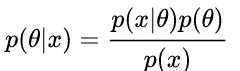为一般性的公式
这里的x是样本，seta是决定样本如何分布的参数
ps 可以把这里的seta理解为原因，x理解为结果，因为seta决定了x是什么样的
解释如下：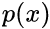evidence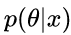后验概率 posterior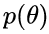先验分布 prior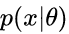似然分布 likelihood

本文与之前的另一篇博客内容相似，可以二者结合
https://blog.csdn.net/qq_40597317/article/details/81002463
https://blog.csdn.net/qq_40597317/article/details/80639989  用到了贝叶斯公式，但是该博文没有详细写明
展开全文• （一）二项分布的最大似然估计  二项分布中，有两个结果：事件要么发生，要么不发生。二项分布中，随机变量X取值1表示事件发生，而取值0表示事件不发生。令p表示事件发生的概率，则(1-p)为事件不发生的概率。如...

在看《机器学习导论》时，碰到多项分布的最大似然估计，一开始一直求不到书中给出的结果，经过一番周折才求出来，现拿出来分享。

（一）二项分布的最大似然估计
二项分布中，有两个结果：事件要么发生，要么不发生。二项分布中，随机变量X取值1表示事件发生，而取值0表示事件不发生。令p表示事件发生的概率，则(1-p)为事件不发生的概率。如公式(1)所示：给定大小为N的独立同分布的样本，二项分布的对数似然函数如公式（2）所示：为了求取该函数的最大值，只需要通过求即可，如下：由此可得到参数p的最大似然估计为：（二）多项分布的最大似然估计
多项分布式在二项式分布的推广。多项分布是指事件有多个状态（K个状态），并且状态之间互斥，设每种状态出现的概率为Pi，并且有。同二项分布，多项分布对应的概率密度函数为：给定大小为N的独立同分布的样本，多项分布的对数似然函数如公式（3）所示：并且满足条件。求公式（3）中函数的最大值，即为求给定约束条件函数的最大值，因此可用拉格朗日乘数法。如公式（4）所示。对公式（4）分别对p1，p2，pk求偏导数有：通过求解方程组（5），可得到参数p的最大似然估计为：完毕。


展开全文• 昨天晚上参加阿里巴巴的实习面试，各种被虐。 伯努利分布的最大似然估计
前言
昨天晚上参加阿里巴巴的实习面试，各种被虐。面试了将近90分钟，才做了3个题，加上项目的介绍。在机器学习方面，问到了一个伯努利分布的最大似然估计的推导，想到逻辑回归的推导就是利用最大似然估计，然后就套用了其推导过程。可能前面被说的有点迷糊了，导致最后也没有完整的推导出来，最失败的一次面试了。
对于阿里的暑期实习面试，其实问得内容还是都比较基础的。准备了很多关于特征工程、集成学习等内容，结果都没有考察到。反而被考的基础知识没有准备的比较清楚。这里对伯努利分布以及其最大似然估计做了一个详细的推导，其它的概率模型可以套用该模版。
祝看到此文章的小伙伴都能找到好的工作…

伯努利分布
伯努利分布，又名0-1分布，是一个离散概率分布。典型的示例是抛一个比较特殊的硬币，每次抛硬币只有两种结果，正面和负面。抛出硬币正面的概率为

p

p

，抛出负面的概率则为

1

−

p

1-p

。因此，对于随机变量

X

X

，则有：

f

(

X

=

1

)

=

p

f

(

X

=

0

)

=

1

−

p

\begin{aligned} f(X=1) &amp; = p \\ f(X=0) &amp; =1-p \end{aligned}

由于随机变量

X

X

只有 0 和 1 两个值，

X

X

的概率分布函数可写为：

(1)

f

(

X

)

=

p

x

(

1

−

p

)

1

−

x

0

&lt;

p

&lt;

1

f(X)=p^x(1-p)^{1-x}\qquad\text{$0&lt;p&lt;1$}\tag{1}

数学期望
在概率论和统计学中，数学期望（或均值）是试验中每次可能结果的概率乘以其结果的总和。它反映了随机变量平均取值的大小。
离散型
离散型随机变量

X

X

的数学期望为一切可能的取值

x

i

x_i

与对应的概率

p

(

x

i

)

p(x_i)

的乘积之和，即如果随机变量的取值为集合

{

x

1

,

x

2

,

⋯
&ThinSpace;

,

x

n

}

\lbrace x_1,x_2,\cdots, x_n \rbrace

，每个取值对应的概率为

{

p

(

x

1

)

,

p

(

x

2

)

,

⋯
&ThinSpace;

,

p

(

x

n

)

}

\lbrace p(x_1),p(x_2),\cdots, p(x_n) \rbrace

，则有：

(2)

E

(

X

)

=

∑

i

=

1

n

x

n

p

(

x

n

)

E(X) =\sum_{i=1}^{n}x_np(x_n) \tag{2}

因此，对于伯努利分布，其数学期望为：

E

(

X

)

=

1

⋅

p

+

0

⋅

(

1

−

p

)

=

p

E(X)=1\cdot p + 0\cdot (1-p)=p

对于随机变量

X

​

X​

，其方差和数学期望的公式满足：

(3)

V

a

r

(

X

)

=

E

(

(

X

−

E

(

X

)

)

2

)

=

E

(

x

2

)

−

[

E

(

x

)

]

2

Var(X)=E((X-E(X))^2)=E(x^2)-[E(x)]^2\tag{3}

一个随机变量的方差就是衡量随机变量和其数学期望之间的偏离程度。

公式推导如下：

V

a

r

(

X

)

=

E

(

(

X

−

E

(

X

)

)

2

)

=

E

(

X

2

−

2

X

⋅

E

(

X

)

+

[

E

(

X

)

]

2

)

=

E

(

X

2

)

−

2

⋅

E

(

X

)

⋅

E

(

X

)

+

[

E

(

X

)

]

2

=

E

(

X

2

)

−

[

E

(

X

)

]

2

\begin{aligned} Var(X)&amp;=E((X-E(X))^2)\\ &amp;=E(X^2-2X\cdot E(X)+[E(X)]^2)\\ &amp;=E(X^2)-2\cdot E(X) \cdot E(X) + [E(X)]^2\\ &amp;=E(X^2)-[E(X)]^2 \end{aligned}

对于伯努利分布，有

E

(

X

2

)

=

E

(

X

)

E(X^2)=E(X)

。因此，其方差为：

V

a

r

(

X

)

=

p

−

p

2

=

p

(

1

−

p

)

Var(X)=p-p^2=p(1-p)

最大似然估计
在统计学中，最大似然估计（MLE），也称为极大似然估计，是用来估计一个概率模型的参数的方法。其目的就是：利用已知的样本结果，反推最有可能导致这样结果的参数值。
由于样本集中的样本都是独立同分布，现以伯努利分布来推导其参数

p

p

最大似然估计。记已知的样本集为：

D

=

{

x

1

,

x

2

,

⋯
&ThinSpace;

,

x

n

}

D=\lbrace x_1,x_2,\cdots,x_n\rbrace

其似然函数为：

(4)

L

(

p

∣

x

1

,

⋯
&ThinSpace;

,

x

n

)

=

f

(

X

∣

p

)

=

f

(

x

1

,

x

2

,

⋯
&ThinSpace;

,

x

n

∣

p

)

=

∏

i

=

1

n

f

(

x

i

∣

p

)

=

∏

i

=

1

n

p

x

i

(

1

−

p

)

1

−

x

i

\begin{aligned} L(p|x_1,\cdots,x_n)&amp;=f(X|p)\\ &amp;=f(x_1,x_2,\cdots,x_n|p)\\ &amp;=\prod_{i=1}^n{f(x_i|p)}\\ &amp;=\prod_{i=1}^n{p^{x_i}(1-p)^{1-x_i}}\tag{4} \end{aligned}

由于有连乘运算，通常对似然函数取对数来计算，即对数似然函数。因此其对数似然函数为：

(5)

L

=

log

⁡

∏

i

=

1

n

f

(

x

i

∣

p

)

=

∑

i

=

1

n

log

⁡

f

(

x

i

∣

p

)

=

∑

i

=

1

n

[

x

i

log

⁡

p

+

(

1

−

x

i

)

log

⁡

(

1

−

p

)

]

\begin{aligned} L&amp;=\log \prod_{i=1}^nf(x_i|p)\\ &amp;=\sum_{i=1}^n{\log f(x_i|p)}\\ &amp;=\sum_{i=1}^n{[x_i\log p+(1-x_i)\log (1-p)]}\tag{5} \end{aligned}

等式

(

5

)

(5)

其实就是逻辑回归中使用到的交叉熵了。

p

^

=

arg

⁡

max

⁡

p

L

(

p

∣

X

)

=

arg

⁡

max

⁡

p

∑

i

=

1

n

[

x

i

log

⁡

p

+

(

1

−

x

i

)

log

⁡

(

1

−

p

)

]

\begin{aligned} \hat{p}&amp;=\arg \max_{p}L(p|X)\\ &amp;=\arg \max_p {\sum_{i=1}^n{[x_i\log p+(1-x_i)\log (1-p)]}} \end{aligned}

因此，最大似然估计其实就是求似然函数的极值点，将对数似然函数对参数

p

p

求导：

∂

L

∂

p

=

∑

i

=

1

n

[

x

i

p

+

1

−

x

i

p

−

1

]

=

∑

i

=

1

n

p

−

x

i

p

(

p

−

1

)

=

0

\begin{aligned} \frac{\partial L}{\partial p}&amp;=\sum_{i=1}^n{[\frac{x_i}{p}+\frac{1-x_i}{p-1}]}\\ &amp;=\sum_{i=1}^n{\frac{p-x_i}{p(p-1)}}=0 \end{aligned}

从而得到伯努利的最大似然估计为：

∑

i

=

1

n

(

p

−

x

i

)

=

0

&ThickSpace;

⟹
&ThickSpace;

p

=

1

n

∑

i

=

1

n

x

i

\begin{aligned} &amp;\sum_{i=1}^{n}{(p-x_i)}=0\\ \implies &amp;p=\frac{1}{n}\sum_{i=1}^n{x_i} \end{aligned}

总结
求概率模型的最大似然估计的一般步骤如下：
写出随机变量的概率分布函数；写出似然函数；对似然函数取对数，并进行化简整理；对参数进行求导，找到似然函数的极值点；解似然方程。
相信了解逻辑回归算法的小伙伴已经看出来了，对逻辑回归的推导其实质上也是最大似然估计算法。在逻辑回归中，其概率分布函数不再是

f

(

x

)

=

p

x

(

1

−

p

)

1

−

x

f(x)=p^x(1-p)^{1-x}

，而是：

(6)

P

(

y

∣

x

;

θ

)

=

(

h

θ

(

x

)

)

y

(

1

−

h

θ

(

x

)

)

1

−

y

P(y|x;\theta)=(h_{\theta}(x))^y(1-h_{\theta}(x))^{1-y}\tag{6}

其中：

(7)

h

θ

(

x

)

=

1

1

+

e

−

z

=

1

1

+

e

−

θ

T

x

h_{\theta}(x)=\frac{1}{1+e^{-z}}=\frac{1}{1+e^{-\theta^{T} x}}\tag{7}

详细过程参见：逻辑回归推导
展开全文机器学习
• L1、L2 正则详解 （解空间、先验分布） 引入 直观看 解空间 先验分布 最大似然估计 最大后验估计L1、L2正则化 最大后验估计 解空间 先验假设
• 一、什么叫先验分布、后验分布似然估计   这几个概念可以用“原因的可能性”和“结果的可能性”的“先后顺序”及“条件关系”来理解。下面举例：   隔壁老王要去10公里外的一个地方办事，他可以选择走路，骑...
• ## 最大似然估计总结笔记

万次阅读 多人点赞 2011-01-09 13:44:00
最大似然估计学习总结------MadTurtle 1. 作用 在已知试验结果（即是样本）的情况下，用来估计满足这些样本分布的参数，把可能性最大的那个参数作为真实的参数估计。 2. 离散型 设为离散型随机变量，为多维参数向量...windows 算法
• 给定数据集D={x1, x2, ... , xn}，假设都服从均值为mean（假设未知），方差为var的高斯分布（假设...最大似然估计（MLE）： MLE过程： （1）假设真实高斯分布的均值为20，方差为18 （2）根据该概率分布抽取100个
• ## 极大似然估计详解

万次阅读 多人点赞 2017-05-28 00:55:10
极大似然估计  以前多次接触过极大似然估计，但一直都不太明白到底什么原理，最近在看贝叶斯分类，对极大似然估计有了新的认识，总结如下： 贝叶斯决策  首先来看贝叶斯分类，我们都知道经典的贝叶斯公式： ...
• 多元正态分布的概率密度函数 N维随机向量 ...多元正态分布的最大似然估计 我们对均值求偏导 针对上面的矩阵求导，给出如下证明： 下面两个是常用的两个公式： 第一个公式证明，和上面的类似。 第个小编
• #极大似然估计 伯努利分布 高斯分布 正态分布 概率分布的参数能以最高的概率产生这些样本。 如果观察到的数据是 D1,D2,D3,...,DND_1, D_2, D_3, ... , D_ND1​,D2​,D3​,...,DN​, 那么极大似然的目标如下： maxP...
• 极值分布的极大似然估计及计算机实现 - read维普资讯第3()卷 第 6期 河北师范大学学报 (自然科学版) V(l3()N0．621)1)6年 11月 JournalofHel,elNormalUniversity(Natural~％ienceEdition) NOV．2006极值分布的极大...
• 文章目录引言概念后验...介绍先验分布/后验分布/似然估计 参考 一个例子搞清楚（先验分布/后验分布/似然估计） 概念 故事： 隔壁小哥要去15公里外的一个公园，他可以选择步行走路，骑自行车或者开辆车，然后通过其...统计学原理
• Day14最大似然估计贝叶斯公式带来的思考定义例子二项分布正态分布 最大似然估计 贝叶斯公式带来的思考 定义 例子 二项分布 正态分布python 机器学习
• 极大似然估计 在参数模型的背景下，标准技术是考虑似然的最大值（或对数似然）。考虑到一些技术性假设，如 ，的某个邻域，那么 其中表示费雪信息矩阵。在此考虑一些样本，来自广义帕累托分布，参数为 ，因此 ...R语言
• 转载于：... 博主用一个例子生动形象的介绍了（先验分布/后验分布/似然估计/贝叶斯公式）. 1、根据结果估计（猜）原因（交通方式）的概率分布即 后验概率：  一般化公式：  P(因|果) 2、根据...
• ## 正态分布均值的极大似然估计

万次阅读 多人点赞 2019-04-04 16:09:53统计学
• 求概率模型的最大似然估计的一般步骤如下： 写出随机变量的概率分布函数； 写出似然函数； 对似然函数取对数，并进行化简整理； 对参数进行求导，找到似然函数的极值点； 解似然方程。 似然函数是一种关于统计模型...
• 极大似然估计法是求估计的另一种方法。它最早由高斯提出。后来为费歇在1912年的文章中重新提出，并且证明了这个方法的一些性质。极大似然估计这一名称也是费歇给的。这是一种上前仍然得到广泛应用的方法。它是建立在...
• 本文原始地址：最大似然估计（Maximum likelihood estimation, 简称MLE）和最大后验概率估计（Maximum a posteriori estimation, 简称MAP）是很常用的两种参数估计方法，如果不理解这两种方法的思路，很模式识别 机器学习 贝叶斯
• 极大似然估计 ... 本篇博客主要讲解以下内容： 一、参数估计 、极大释然估计 1．似然函数 2．极大似然估计（MLE） ...5．求极大似然估计的一般步骤 ...三、常见分布的参数估计 1．高斯分布 ...3．二项分布（Bi...参数估计
• 正态分布密度函数是： 　若随机变量X服从一个数学期望为μ、方差为σ2的正态分布，记为N(μ，σ2)。当μ=0，σ2=1是，称为标准正态分布。不需要记住这个复杂的...最大似然估计量 　随机变量X服从正态分布：
• 正态分布被命名为高斯分布，我们也容易认为是高斯发现了正态分布，其实不然，高斯分布最早由棣莫弗在1718年著作的书籍（Doctrine of Change），及1734年发表的一篇关于二项分布文章中提出的，不过高斯对于正态分布...统计学
• 这个多项式分布的参数，采用极大估计是怎么求的呢?当时想了想还真不知道，于是在网上找了资料，学习了一下，特此记录。公式推导很多情况下，假定一个变量XX有kk个状态，其中k&gt;2k&gt;2,每个状态假定的可能...
• 通过样例来讲解最大似然估计和极大似然估计以及他们的区别人工智能 机器学习 算法
• ## 多元正态分布的极大似然估计

万次阅读 多人点赞 2018-06-20 13:53:12
多元正态分布的极大似然估计 1. 一元正态分布的密度函数 一元正态分布的密度函数表示为： f(x)=1(2π)−−−−√σe−(x−μ)22σ2f(x)=1(2π)σe−(x−μ)22σ2f(x) = \frac{1}{\sqrt {(2 \pi)} \sigma} e^{...
• 我们在前面的章节中见识过维正态分布，(X,Y)服从参数为μ1, μ2, σ1, σ2, ρ的维正态分布，记作(X, Y)~N(μ1, μ2, σ1, σ2, ρ)，它的密度函数： 　其中μ1是第1维度的均值，σ12是第1维度的方差，ρ是将...
• 2二项分布，也叫做n重伯努利分布 参考链接2 两者的区别来自知乎 参考链接3 3.均匀分布的参数估计 参考链接4 均匀分布的参数估计 参考链接 就是这么多数的最大值和最小值，分别就是他的a和b 4.正太分布用numpy生成的......